×#### Thank you for registering.

One of our academic counsellors will contact you within 1 working day.

Click to Chat

1800-1023-196

+91-120-4616500

CART 0

• 0

MY CART (5)

Use Coupon: CART20 and get 20% off on all online Study Material

ITEM
DETAILS
MRP
DISCOUNT
FINAL PRICE
Total Price: Rs.

There are no items in this cart.
Continue Shopping• Complete Physics Course - Class 11
• OFFERED PRICE: Rs. 2,968
• View Details

Work-Energy Theorem

Table of Content

Body Under the Action of an Unbalanced Force

Constant Force

Body Under the Action of a Balanced Force

Conservation of Mechanical Energy

Conservative Force (F)

Non-Conservative Force

Solved Problems

Related Resources

It states that work done on/by a body gets converted into energy.

The type of energy depends upon the nature of force / forces acting on it.(a) Body Under the Action of an Unbalanced Force

Work-Energy principle for a body, under the action of an unbalanced force, can be stated as follows:

Constant Force

It states that, “The net work done (Wnet) by the forces acting on a body is equal to the change in the kinetic energy of the body”.

So, Wnet = ½ mv2 – ½ mu2

= Kf – Ki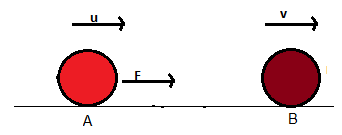If work is done on the body, its K.E increases and if work is done by the body, its K.E. decreases.

Consider a force F acting on a moving body. As a result of this, the body moves from A to B and gets its velocity increased from u to v as shown in the below figure.

If dW is the work done in moving through a small distance ‘ds’,

So, dW = F ds cos 0º

= F ds

= ma ds

Here ‘a’ is the acceleration of the body.

Since, a = m(dv/dt)

So, dW = [m(dv/dt)].ds

= m dv (ds/dt)

dW = mv dv

Integrating both sides,

[W-0] = m[v2/2]

or, W = m[v2/2 – u2/2]

or, W = ½ mv2 – ½ mu2 = Final K.E – Initial K.E

Thus, Work done = Change in kinetic energy

Therefore, the change in kinetic energy of a body equals the total work done by all the forces (conservative and non-conservative).

This is in accordance with the law of conservation of energy.

(b) Body Under the Action of a Balanced Force

Work done on/by a body shall result in a change of kinetic energy of the body if the force acting on it is unbalanced. The body may move under the action of the balanced force.

In that case:

(i) there shall be no acceleration in the body

(ii) there shall be no change in kinetic energy

(iii) work done on the body by the applied force shall be converted into the potential energy which, in turn, shall result in change of position or configuration of the body.

Conservation of Mechanical EnergyMechanical energy of a system is the sum total of its kinetic and potential energy.

It states that, ”sum total of energy (of all kinds) of a system always remains constant while conservation of energy from one form to another may take place continuously.

An interesting case of the work-energy theorem occurs when all the forces acting on a body are conservative. In this case, one can define a potential energy for each of these forces: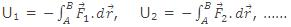where A and B are initial and final positions, respectively.

The work-energy theorem can now be re-written by using the relations,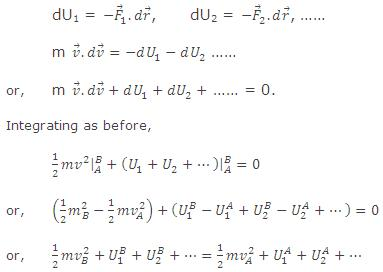This result states that the total mechanical energy,

Etotal = Ekinetic + Epotential = 1/2mv2 + U1 + U2 + ... is conserved.

Hence, Ekinetic + Epotential = constant.

Thus, if non-conservative forces are not present or if these forces are present but do not do any work, the mechanical energy of the system remains constant.

On a roller coaster, energy is transformed from potential energy to kinetic energy and vice versa. Provided that external forces (such as friction forces and applied forces) do not do work, the total amount of mechanical energy will be held constant.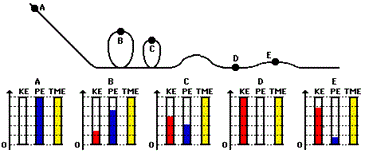The motion of the slider in the animation below is similar to the motion of a roller coaster car on a roller coaster track. The similarity of the two motions is associated with the work-energy relationship. The energy bar charts accompanying the animation depict this relationship. Energy bar charts are a conceptual tool amount the amount of each form of energy possessed by an object as it an undergoes a particular motion. Observe from the animation that the total mechanical energy (TME) of the slider remains constant throughout the motion. The total mechanical energy is the sum of the two forms of mechanical energy - kinetic energy (KE) and potential energy (PE). While the individual amounts of kinetic and potential energy are undergoing change, their sum will always be the same amount.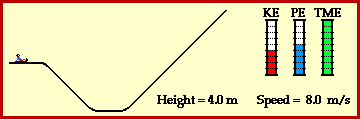Conservative Force (F)

Conservative force is equal to the negative gradient of potential V of the field of that force. This force is also called central force.

So,  F = - (dV/dr)

The line integral of a conservative force around a closed path is always zero.

So,

Non-Conservative Force

Force is non-conservative if the work done by that force on a particle that moves between two points depends on the path taken between these points.

Refer this video for better understanding about conservation of energy:-

Solved Problems

Problem 1:-

A proton (nucleus of the hydrogen atom) is being accelerated in a linear accelerator. In each stage of such an accelerator the proton is accelerated along a straight line 3.60×1015 m/s2. If a proton enters such a stage moving initially with a speed of 2.40×107 m/s and the stage is 3.50 cm long, compute (a) its speed at the end of the stage and (b) the gain in kinetic energy resulting from the acceleration. The mass of the proton is 1.67×10-27 kg. Express the energy in eV.

Concept:-

Kinetic energy K of a body having mass m moving with speed v is equal to the ½ mv2.

So, K = ½ mv2    …… (1)

From equation (1), speed of the particle will be,

v = √2K/m       …… (2)

Work done (W) by a force F that moves a body through a displacement x will be,

W = Fx          …… (3)

Again in accordance to work energy theorem, the net work done by the forces acting on a body is equal to the change in the kinetic energy ΔK of the body.

So, ΔK = Kf- Ki

= W           …… (4)

From equation (3) and (4) we get,

ΔK = W

= Fx

= max           (Since, F = ma) …… (5)

Solution:-

?(a) To obtain the final speed vf of the proton, first we have to find out the final kinetic Kf energy of the proton.

To obtain the change in the kinetic energy ΔK, substitute 1.67×10-27 kg for mass of the proton m, 3.60×1015 m/s2 for a and 3.50 cm for x in the equation  ΔK = max, we get,

ΔK = max

= (1.67×10-27 kg) (3.60×1015 m/s2)( 3.50 cm) = (1.67×10-27 kg) (3.60×1015 m/s2)( 3.50 cm×10-2 m/1 cm)

=(2.10×10-13 kg. m2/s2) (1 J/1 kg. m2/s2)  = (2.10×10-13 J) (1 eV/1.6×10-19 J) = 1.31×106 eV

So, Kf- Ki = 2.10×10-13 J (Since, ΔK = Kf- Ki)

Or, Kf = (2.10×10-13 J) + Ki

= (2.10×10-13 J) + ½ mvi2= (2.10×10-13 J) + ½ (1.67×10-27 kg) (2.40×107 m/s2)2

= [2.10×10-13 J] +[(4.81×10-13 kg. m2/s2) (1 J/1 kg. m2/s2)]= [2.10×10-13 J] +[4.81×10-13 J]

=6.91 ×10-13 J

To obtain the final speed vf of the proton, substitute 6.91 ×10-13 J for Kf and 1.67×10-27 kg for mass of the proton m in the equation v = √2Kf/m, we get,

v = √2Kf/m =√2 (6.91 ×10-13 J) /(1.67×10-27 kg)  (1 kg. m2/s2/ 1 J)

=2.88×107 m/s

From the above observation we conclude that, the final speed vf of the proton would be 2.88×107 m/s.

(b) To find out the speed of proton at the end of the stage, we have to find out the change in the kinetic energy ΔK.

To obtain the change in the kinetic energy ΔK, substitute 1.67×10-27 kg for mass of the proton m, 3.60×1015 m/s2 for a and 3.50 cm for x in the equation  ΔK = max, we get,

ΔK = max

= (1.67×10-27 kg) (3.60×1015 m/s2)( 3.50 cm) = (1.67×10-27 kg) (3.60×1015 m/s2)( 3.50 cm×10-2 m/1 cm)

=(2.10×10-13 kg. m2/s2) (1 J/1 kg. m2/s2) = (2.10×10-13 J) (1 eV/1.6×10-19 J)

= 1.31×106 eV

From the above observation we conclude that, the speed of proton at the end of the stage would be 1.31×106 eV.

Problem 2:-

A 3700-lb automobile (m = 1600 kg) starts from rest on a level road and gains a speed of 45 mi/h (= 72 km/h) in 33 s. (a) What is the kinetic energy of the auto at the end of the 33s? (b) What is the average net power delivered to the car during the 33-s interval? (c) What is the instantaneous power at the end of the 33-s interval assuming that the acceleration was constant?

Concept:-

Kinetic energy K of a body having mass m moving with speed v is equal to the ½ mv2.

So, K = ½ mv2       …… (1)

The power (P) due to the force acting on a body is equal to the work done on the body (W) divided by time (t).

Power delivered (P) to the body is equal to the force acting on the body (F) times speed of the body (v).

So, P = W/t           …… (2)

So, P = Fv     …… (3)

But force acting on the body (F) is equal to the mass of the body (m) times acceleration of the body (a).

F = ma       …… (4)

To obtain the power (P) in terms of mass (m) and acceleration (a), substitute ma for F in the equation P = Fv,

P = Fv

= mav      …… (5)

Acceleration (a) is the rate of change of velocity (v).

So, a = v/t     …… (6)

Substitute the value of a in the equation (5), the power (P) will be,

P = mav

= m (v/t) v = mv2/t          …… (7)

Solution:-

(a) We have to find out the kinetic energy of the auto having mass 1600 kg and at a speed of 72 km/h.

To obtain Kinetic energy K of the auto, substitute 1600 kg for mass m and 72 km/h for speed the football v in the equation K = ½ mv2,

K = ½ mv2

= ½ (1600 kg) (72 km/h)2

=½ (1600 kg) ((72 km/h)(103 m/1 km) (1 h/3600 s)) 2= (3.2×105 kg. m2/s2) (1 J/1 kg. m2/s2)

= 3.2×105 J     …… (8)

From equation (8) we observed that, the kinetic energy of the auto will be 3.2×105 J.

(b) We have to find out the average net power delivered to the car during the 33 s interval.

To obtain the net power (P) of the auto, substitute 3.2×105 J for kinetic energy K and 33 s for the time t in the equation P = W/t,

P = W/t

= (3.2×105 J)/33 s = (9.7×103 J/s) (1 W / 1 J/s)

= 9.7×103 W     …… (9)

From equation (9) we observed that, the average net power delivered to the car during the 33 s interval will be 9.7×103 W.

(c) We have to find out the instantaneous power at the end of the 33 s interval assuming that the acceleration was constant.

To obtain power (P), substitute 1600 kg for mass m,  and 72 km/h for speed v and 33 s for time t in the equation P = mv2/t,

P = mv2/t

= (1600 kg) (72 km/h)2/33 s

= (1600 kg) ((72 km/h)(103 m/1 km) (1 h/3600 s)) 2 /33 s = (1.9×104 kg. m/s3) (1 W/1 kg. m/s3)

= 1.9×104 W         …… (10)

From equation (10) we observed that, the instantaneous power at the end of the 33 s interval will be 1.9×104 W.

Problem 3:-

The string in below figure has a length L = 120 cm, and the distance d to the fixed peg is 75.0 cm. When the ball is released from rest in the position shown, it will follow the arc shown in the figure. How fast will it be going (a) when it reaches the lowest point in its swing and (b) when it reaches its highest point, after the string catches on the peg?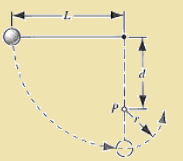Concept:-

Kinetic energy K of an object is defined as,

K = ½ mv2

Here m is the mass of the object and v is the speed of the object.

Potential energy U is defined as,

U = mgy

Here, m is the mass of the object, g is the free fall acceleration and y is the height.

In accordance to conservation of energy, the total change in kinetic energy  ?Ktoatal of all the particles that make up the system is equal in magnitude, but opposite in sign, to the total change in the potential energy -?Utotal of the system.

?Ktoatal = -?Utotal

So,

K-K0= -(U-U0)

Solution:-

Assume that, U0= K0 = 0

Substitute 0 for U0 and 0 for K0 in the equation K-K0= -(U-U0), we get,

K-0 = -(U-0)

So, K= -U

Substitute ½ mv2 for kinetic energy K and mgy for potential energy U in the equation

K= -U, we get,

K= -U

½ mv2= -mgy

v2/2 = -gy

Or, v = √2g(-y)

(a) To obtain the speed v of the ball, when it reaches the lowest point in its swing, substitute 9.81 m/s2 for free fall acceleration g and  -120 cm for y (negative sign for negative axis) in the equation v = √2g(-y), we get,

v = √2g(-y)

=√2(9.81 m/s2)(-(-120 cm))

=√2(9.81 m/s2)(120 cm)(10-2 m/1cm)

=4.85 m/s

From the above observation we conclude that, the speed v of the ball, when it reaches the lowest point in its swing would be 4.85 m/s.

(b) The value of y, when the ball reaches its highest point would be,

y = -[120 cm-(120 cm-75.0 cm)-(120 cm-75.0 cm)]

= (- 30 cm) (10-2 m/1 cm)

= -0.30 m

To obtain the speed v of the ball, when the ball reaches its highest point, substitute 9.81 m/s2 for free fall acceleration g and  -0.30 m for y (negative sign for negative axis) in the equation v = √2g(-y), we get,

v = √2g(-y)

=√2(9.81 m/s2)(-(-0.30 m))

=2.43 m/s

From the above observation we conclude that, the speed v of the ball, when the ball reaches its highest point, after the string catches on the peg would be 2.43 m/s.

Related Resources

You might like to refer Kinetic Energy

For getting an idea of the type of questions asked, refer the Sample Papers of Previous Years.### Course Features

• 101 Video Lectures
• Revision Notes
• Previous Year Papers
• Mind Map
• Study Planner
• NCERT Solutions
• Discussion Forum
• Test paper with Video Solution